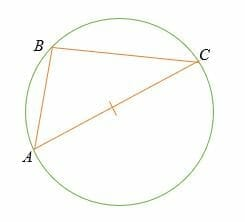## What is the Thales Theorem?

### Applications of Thales Theorem

In geometry, none of the topics is without any real-life use. Therefore, Thales Theorem also has some applications:

• We can accurately draw a tangent to a circle using Thales Theorem. You can use a set square for this purpose.
• We can accurately find the centre of the circle using Thales Theorem. The tools used for this application are a set the square and sheet of paper. Firstly, you have to place the angle at the circumference. The intersections of two points with circumference state the diameter. You can repeat this using a different pair of points, which will give you another diameter. The intersection of diameters will give you the centre of the circle.

The Thales theorem states that:

If three points A, B and C lie on the circumference of a circle, whereby the line AC is the diameter of the circle, then the angle ABC is a right angle (90°).

Alternatively, the Thales theorem can be stated as:

The diameter of a circle always subtends a right angle to any point on the circle.You noticed that the Thales theorem is a special case of the inscribed angle theorem (the central angle = twice the inscribed angle).

Thales theorem is attributed to Thales, a Greek mathematician and philosopher who was based in Miletus. Thales first initiated and formulated the Theoretical Study of Geometry to make astronomy a more exact science.

There are multiple ways to prove Thales Theorem. Both geometry and algebra techniques can be used to prove this theorem.